# Stochastic equivalence

(diff) ← Older revision | Latest revision (diff) | Newer revision → (diff)
The equivalence relation between random variables that differ only on a set of probability zero. More precisely, two random variables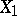and, defined on a common probability space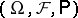, are called stochastically equivalent if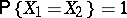. In most problems of probability theory one deals with classes of equivalent random variables, rather than with the random variables themselves.
Two stochastic processes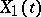and,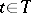, defined on a common probability space are called stochastically equivalent if for anystochastic equivalence holds between the corresponding random variables: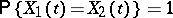. With regard to stochastic processesand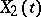with coinciding finite-dimensional distributions, the term "stochastic equivalence" is sometimes used in the broad sense.